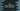# Java program to clear a vector or delete all elements of a vector# Introduction :

In this post, we will learn how to clear a vector in Java. A vector is like a dynamic array in Java. It doesn’t have any fixed length. We can add as many items as we want to a vector.

Clearing a vector means deleting all elements from a vector. It will not delete the vector object. It will only remove all elements from the vector. To check if a vector is cleared, we will confirm it by checking the size of the vector. If the size is 0, it means that the vector is cleared.

Our program will ask the user to enter the total count of the vector. It will then take the inputs for each element from the user one by one. The program will also print out the vector to the user before clearing the elements. After clearing the inputs, it will print out the vector one more time. It will also print the size of the vector on each step.

Let’s take a look at the program first :

## Java program to create and clear a vector :

``````import java.util.Scanner;
import java.util.Vector;

public class Example {
public static void main(String[] args) {
//1
Vector vector = new Vector<>();
int size;
Scanner sc = new Scanner(System.in);

//2
System.out.println("Enter the size of the vector : ");
size = sc.nextInt();

//3
for (int i = 0; i < size; i++) {
System.out.println("Enter value for position " + (i + 1) + " : ");
}

//4
System.out.println("You have entered : " + vector);
System.out.println("Size of the vector is : " + vector.size());

//5
vector.clear();

//6
System.out.println("After the vector is cleared : " + vector);
System.out.println("Size of the vector after cleared : " + vector.size());
}
}``````

### Explanation :

The commented numbers in the above program denote the step numbers below :

1. Create one Vector variable named vector. This variable can hold only integer numbers. Create one integer variable size to hold the size of the vector. Also, create one scanner variable to get the user inputs.
2. Ask the user to enter the size of the vector. Read it using the scanner variable and save it in the variable size.
3. Use one_ for loop_ to take the user inputs for the vector. This loop will run for size number of times. On each iteration of the loop, ask the user to enter the value for that specific position. Using the scanner variable, read the user input and add it to the vector. For adding a new value to the vector, we are using ‘add()’ method. For reading the user input integer, we are using the ‘nextInt() method. Note that this program will work only with integer inputs. If the user inserts anything other than integer value, it will crash.
4. Print out the complete vector that the user has entered on the previous steps. Also, print the size of the vector. For printing the size, we are using size() method.
5. This step is used for clearing the vector. The clear() method is used to clear the vector.
6. After the vector is cleared, the program is printing out the final vector variable again. It is also printing the size of the vector.

### Sample Output for the above clear vector Java program :

``````Enter the size of the vector :
3
Enter value for position 1 :
1
Enter value for position 2 :
2
Enter value for position 3 :
3
You have entered : [1, 2, 3]
Size of the vector is : 3
After the vector is cleared : []
Size of the vector after cleared : 0

Enter the size of the vector :
4
Enter value for position 1 :
4
Enter value for position 2 :
12
Enter value for position 3 :
23
Enter value for position 4 :
34
You have entered : [4, 12, 23, 34]
Size of the vector is : 4
After the vector is cleared : []
Size of the vector after cleared : 0``````

### Conclusion :

As you have seen, we can easily clear one vector in Java using the_ clear()_ method. It is a good practice to use the same object by clearing the data instead of using a different one. Try to run the program on your PC and drop one comment below if you have any queries.